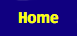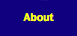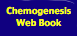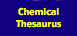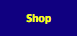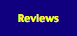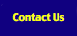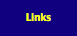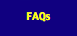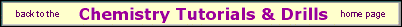Stoichiometry and Balancing Chemical Equations

The ability to balance chemical equations in terms of mass and charge is one of the key skills that must be mastered by the serious student of chemistry.

We believe that the best way of learning how to balance chemical equations with confidence is through practice.

On this web site, there are 1270 chemical reactions pulled from The Chemical Thesaurus reaction chemistry database that you can use to test and improve your equation balancing skills. The drill problems range from trivial to really very difficult. We expect that everybody will find the Expert Drill problems challenging.

Our reaction equation balancing drills:

171 Trivial & Easy reaction equation balancing problems

574 Intermediate reaction equation balancing problems

23 Expert reaction equation balancing problems

253 Reaction equation balancing problems from A-Level Chemistry (AS)

249 Reaction equation balancing problems from A-Level Chemistry (A2)

We recommend the following web pages as preparation for the reaction equation balancing drills problems presented here:

Stoichiometry from the Wikipedia

Stoichiometry from the UNC-Chapel-Hill Chemistry Fundamentals Program.

Equation Balancing & Stoichiometry Lectures on the Chembuddy website

Stoichiometry search on gooooogle

Queries, Suggestions, Bugs, Errors, Typos...

If you have any:

Queries